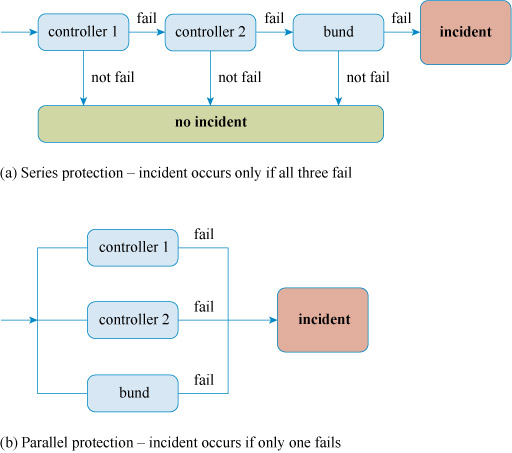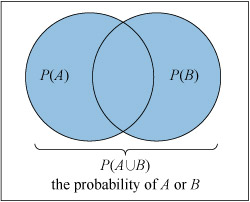Science, Maths & Technology

### Become an OU studentAssessing risk in engineering, work and life

Start this free course now. Just create an account and sign in. Enrol and complete the course for a free statement of participation or digital badge if available.

# Series and parallel

So far, these calculations have looked at the probability of several events all happening. For instance, in the situation described above, a leak would occur only if both detectors failed and the bund failed. In effect, the control systems are working in series (Figure 16a). However, in other instances, an undesired outcome might take place if any one of a number of events occurred. In such a case, the control systems would be working in parallel (Figure 16b). For example, a house could be burgled if either the door was left unlocked or a ground-floor window was left open.Figure 16 Controls in series and parallel

## Probability of at least one event occurring

The method for calculating the probability of one or more of a number of events occurring is to calculate the probability of none of the events occurring and then subtract this answer from 1.

For the special case of either or both of two events occurring (A or B), the probability is given by

In probability notation, this is expressed as

where the symbol denotes OR.

Note that the probability of both events occurring has to be deducted to avoid double counting. When the probability of occurring is calculated, those occasions when and both occur are also included. Similarly, when the probability of occurring is calculated, the probability of both and occurring is included. Deducting one of these eliminates this double counting, as shown in the probability space diagram in Figure 17.Figure 17 Probability space diagram for